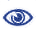# Prague Stringology Conference 2013

Deciding the Density Type of a Given Regular Language

 Abstract: In this paper, the density of a language is the function that returns, for each n, the number of words in the language of length n. We consider the question of deciding whether the density of a given regular language L is exponential or polynomial. This question can be answered in linear time when L is given via a DFA. When L is given via an NFA, we show that L has exponential density if and only if the NFA has a strongly connected component (SCC) in which two equal length walks from the same state have different labels. This characterization leads to a simple quadratic time algorithm. However, a more elegant approach produces a linear time algorithm whose proof of correctness involves the theorem of Fine and Wilf and the greatest common divisor (gcd) of the lengths of all cycles in the SCC. We have implemented both the quadratic and linear time algorithms using the FAdo library for automata, and present results of a few test cases.

 Download paper:PostScript PDF BibTeX reference
 Download presentation: